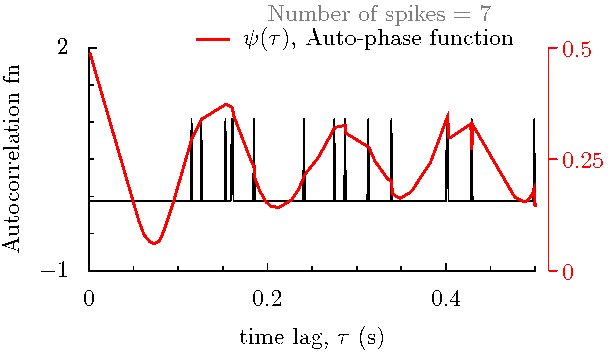About Online computation C++ Class Mathematica Package Matlab function R function
Sweep through data to visualize inherent signal:
• Example: The first 10 data points that are shown in the figure below look like this: {52.4815, 52.6634, 52.838, 53.0339, 53.2145, 53.4398, 53.6131, 53.7865, 53.9781, 54.1936}. Vertical lines are drawn to mark these event times. (The dataset itself is given here.) Below we show the signal recovered from these and subsequent data points as we sweep through the data.

(The flash video may be controled with right mouse click menu)

• A Mathematica notebook showing the above animation by sweeping through the data. (Please run it to see animation.)
Phase function compared with auto-correlation function:
This animation compares the phase function with autocorrelation function computed with number of data points from as few as 3 to as many as 673. The phase function performs better than correlation function for fewer data ponits, and corresponds well with the correlation function for large number of data points.

The data consists of discrete spike time events (given here in seconds) from in vitro recordings of rat globus pallidus neurons. Each comparison is for the selected number of data points (spike time events) shown in "Number of spikes" in the figure. The auto-correlation function is plotted in black, and the auto-phase function is plotted in red.
 <>